# Finding Extrema

Derivatives can be used to find a function's local extreme values, its peaks and valleys.

This post is part of a series.

Derivatives can be used to find a function’s local extreme values, its peaks and valleys. At its peaks and valleys, a function’s derivative is either $0$ (a smooth, rounded peak/valley) or undefined (a sharp, pointy peak/valley).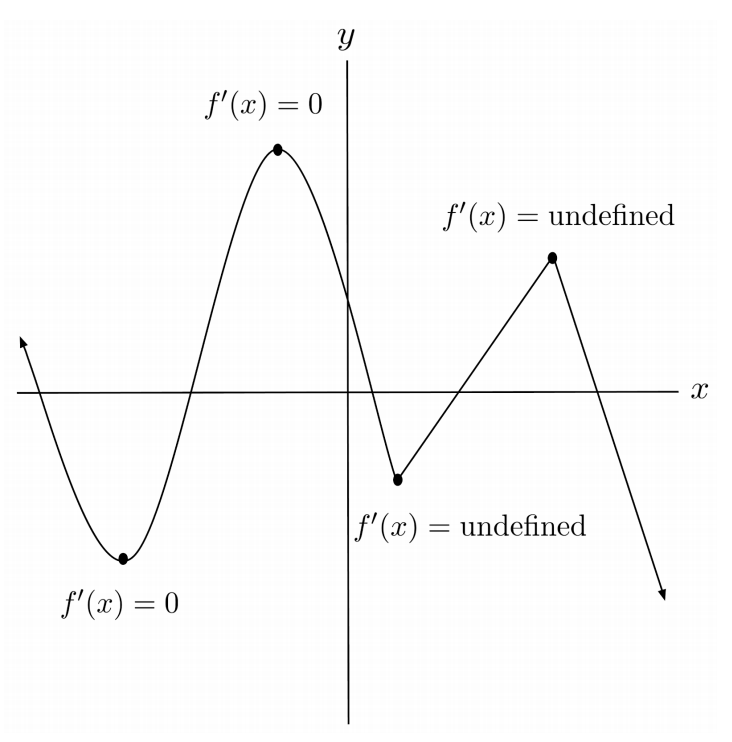## Critical Points

The points at which a function’s derivative is $0$ or undefined, and the function itself exists, are called critical points of the function. We can find the critical points by taking the derivative, noting any singularities, setting the derivative to $0$, and solving.

For example, to find the critical points of the function $f(x)=x \sqrt{1-x^2}$, we start by taking the derivative and simplifying.

\begin{align*} f(x)&=x \sqrt{1-x^2} \\ f'(x)& = \sqrt{1-x^2} - \frac{x^2}{\sqrt{1-x^2}} \\ &= \frac{1-2x^2}{\sqrt{1-x^2}} \end{align*}

The derivative has a singularity when the denominator $\sqrt{1-x^2}$ is $0$, which happens at $x=\pm 1$. The derivative itself is zero when the numerator $1-2x^2$ is $0$, which happens at $x=\pm \sqrt{ \frac{1}{2} }$. The function is defined at all of these x-values, so they all correspond to critical points: $x= \pm 1, \pm \sqrt{ \frac{1}{2} }$.

## Classifying Critical Points

Now, how do we tell which critical points correspond to maxima (peaks), and which correspond to minima (valleys)?

It may be tempting to decide whether a critical point is a maximum or minimum by observing whether the resulting function value is large or small. However, it is entirely possible that some local minima may be greater than some local maxima. Think of a mountain range – some valleys may be higher than some peaks.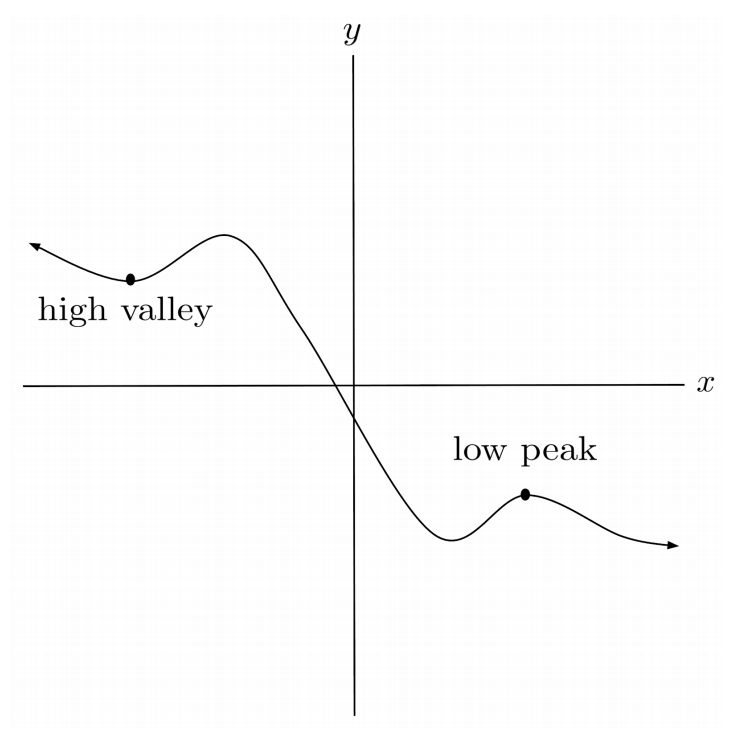It may also be possible that some critical points are neither peaks nor valleys, but saddle points on the side of a mountain where the terrain is flat. At saddle points like the one indicated below, the derivative is $0$ but the point is neither a maximum nor a minimum.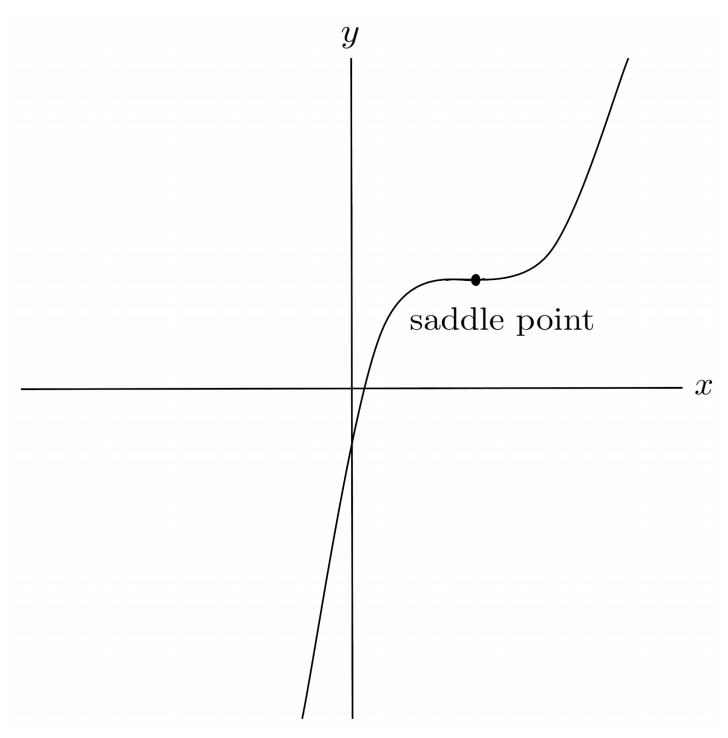## First Derivative Test

There are two main methods for determining whether a critical point is a local minimum, local maximum, or neither. One way is to inspect the sign of the derivative on either side of the critical point, which tells whether we are ascending or descending on either side of the critical point.

• • If the derivative is positive to the left of the critical point and negative to the right of the critical point, then we are ascending to a peak and then descending down the peak, which tells us that the critical point is a local maximum.
• • On the other hand, if the derivative is negative to the left of the critical point and positive to the right of the critical point, then we are descending down a valley and then climbing up the valley, which tells us that the critical point is a local minimum.
• • Lastly, if the derivative does not switch sign from the left of the critical point to the right of the critical point, then we are either ascending up the whole way or descending down the whole way, which indicates that the critical point is a saddle point.

This method is called the first derivative test, because it makes use of the first derivative of the function.

## Demonstration of First Derivative Test

To use the first derivative test on the critical points $x=\pm 1, \pm \sqrt{ \frac{1}{2} }$ that we found for the function $f(x)=x \sqrt{1-x^2}$, we first split up the number line over the critical points.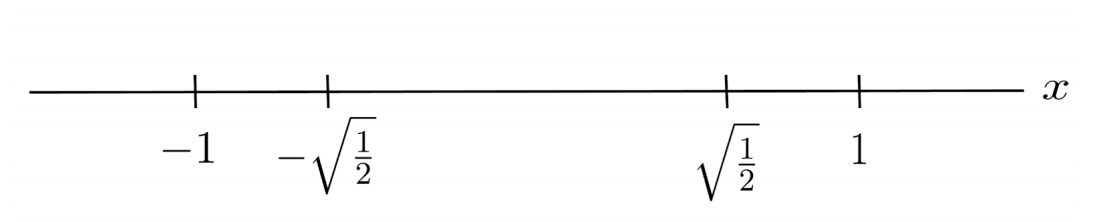The number line splits into $5$ intervals:

\begin{align*} (-\infty,-1) \cup \left( -1,-\sqrt{\frac{1}{2}} \right) \cup \left( -\sqrt{\frac{1}{2}}, \sqrt{\frac{1}{2}} \right) \cup \left( \sqrt{\frac{1}{2}}, 1 \right) \cup (1,\infty) \end{align*}

However, on the intervals $(-\infty,1)$ and $(1,\infty)$ our function $f(x)=x\sqrt{1-x^2}$ is not defined because the argument of the square root becomes negative. We remove these intervals from consideration.

\begin{align*} \left( -1,-\sqrt{\frac{1}{2}} \right) \cup \left( -\sqrt{\frac{1}{2}}, \sqrt{\frac{1}{2}} \right) \cup \left( \sqrt{\frac{1}{2}}, 1 \right) \end{align*}

We want to know whether our function is increasing or decreasing on each of these intervals. To find out this information, we choose a test value in each of the remaining intervals. The actual values of the test values don’t matter, because the derivative maintains the same sign within any given interval. For the sake of example, we choose our test values as, say, $-0.9$, $0$, and $0.9$.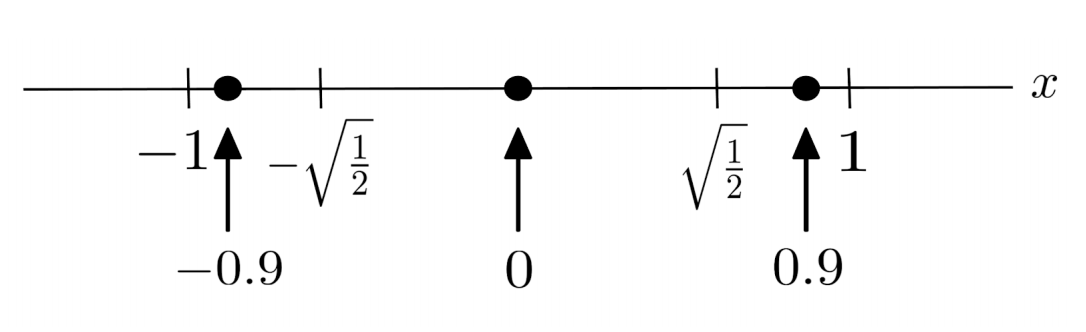Lastly, we evaluate the sign of the derivative at each of these test values.

\begin{align*} f'(x) &= \frac{1-2x^2}{\sqrt{1-x^2}} \\ f'(-0.9) &= \frac{1-2(-0.9)^2}{\sqrt{1-(-0.9)^2}} = \frac{-}{\sqrt{+}} = - \\ f'(0) &= \frac{1-2(0)^2}{\sqrt{1-(0)^2}} = \frac{+}{\sqrt{+}} = + \\ f'(0.9) &= \frac{1-2(0.9)^2}{\sqrt{1-(0.9)^2}} = \frac{-}{\sqrt{+}} = - \end{align*}

The sign of the derivative at each particular test value tells us the sign of the derivative throughout the interval containing the particular test value. As a result, we know whether the function is increasing or decreasing on each interval, and we can sketch a rough graph of the peaks and valleys of the function.We see that the function $f(x)=x\sqrt{1-x^2}$ has maxima at $x=-1,\sqrt{ \frac{1}{2} }$ and minima at $x=-\sqrt{ \frac{1}{2} }, 1$.

## Second Derivative Test

The other method for classifying a critical point of a function as a maximum or minimum is called the second derivative test, because it makes use of the second derivative of the function.

• • If the second derivative is positive at the critical point, then the function is concave up in the shape of a smile, which means the critical point is a local minimum.
• • If the second derivative is negative at the critical point, then the function is concave down in the shape of a frown, which means the critical point is a local maximum.
• • If the second derivative is $0$ or undefined at the critical point, then we cannot conclude whether the critical point is a local maximum or minimum, and we need to fall back to the first derivative test.

The second derivative test is sometimes inconclusive, but it is mentioned because it is often faster than the first derivative test.

## Demonstration of Second Derivative Test

To use the second derivative test on the critical points $x=\pm 1, \pm \sqrt{ \frac{1}{2} }$ that we found for the function $f(x)=x\sqrt{1-x^2}$, we first take the second derivative of the function. We computed the first derivative earlier, so we just have to differentiate once more.

\begin{align*} f'(x) &= \frac{1-2x^2}{\sqrt{1-x^2}} \\ f''(x) &= \frac{-4x\sqrt{1-x^2}+ \frac{x(1-2x^2)}{\sqrt{1-x^2}} }{1-x^2} \\ &= \frac{-4x(1-x^2)+ x(1-2x^2) }{(1-x^2)^\frac{3}{2}} \\ &= \frac{2x^3-3 x}{(1-x^2)^\frac{3}{2}} \end{align*}

We evaluate the sign of the second derivative at each of the critical points.

\begin{align*} f''(-1) &= \frac{-2+3}{(1-1)^\frac{3}{2}} = \frac{+}{0} = \mbox{undefined} \\ f'' \left( - \sqrt{\frac{1}{2}} \right) &= \frac{ \sqrt{\frac{1}{2}} +3 \sqrt{\frac{1}{2}} }{\left( 1- \frac{1}{2} \right)^\frac{3}{2}} = \frac{+}{+} = + \\ f'' \left( \sqrt{\frac{1}{2}} \right) &= \frac{ \sqrt{\frac{1}{2}} -3 \sqrt{\frac{1}{2}} }{\left( 1- \frac{1}{2} \right)^\frac{3}{2}} = \frac{-}{+} = - \\ f''(1) &= \frac{2-3}{(1-1)^\frac{3}{2}} = \frac{-}{0} = \mbox{undefined} \end{align*}

Based on the results of the second derivative test, we see that $x=-\sqrt{ \frac{1}{2} }$ is a minimum, and $x=\sqrt{ \frac{1}{2} }$ is a maximum. The test is inconclusive for $x=-1$ and $x=1$, so we would need to fall back to the first derivative test for these cases.

## When to Use Each Test

In general, it’s a good idea to use the first derivative test when the second derivative is more complex than the first derivative, and the second derivative test when the second derivative is less complex than the first derivative.

For example, for polynomial functions, it is usually easiest to use the second derivative test because the second derivative is less complex than the first derivative.

\begin{align*} p(x) &= 2x^3-3x^2-11x+6 \\ p'(x) &= 6x^2 - 6x - 11 \\ p''(x) &= 12x-6 \end{align*}

We find the critical points by solving for where the first derivative is zero.

\begin{align*} 0 &= 6x^2 - 6x - 11 \\ x &= \frac{3 \pm 5\sqrt{3}}{6} \end{align*}

Then, we find the sign of the second derivative at these points.

\begin{align*} p''\left( \frac{3 - 5\sqrt{3}}{6} \right) &= 12 \left( \frac{3 - 5\sqrt{3}}{6} \right) -6 = 2(3 - 5\sqrt{3})-6 = -10 \sqrt{3} < 0 \\ p''\left( \frac{3 + 5\sqrt{3}}{6} \right) &= 12 \left( \frac{3 + 5\sqrt{3}}{6} \right) -6 = 2(3 + 5\sqrt{3})-6 = 10 \sqrt{3} > 0 \end{align*}

The critical point $x=\frac{3-5\sqrt{3}}{6}$ has a negative second derivative, which means the function is concave down and thus the critical point is a maximum. Likewise, the critical point $x=\frac{3+5\sqrt{3}}{6}$ has a positive second derivative, which means the function is concave up and thus the critical point is a minimum.

On the other hand, for the function below, it is easiest to use the first derivative test because the computations for the second derivative will get a bit messy when we use the product rule.

\begin{align*} f(x) &= e^{x^2 + \frac{1}{x}} \\ f'(x) &= \left( 2x - \frac{1}{x^2} \right) e^{x^2 + \frac{1}{x} } \end{align*}

We find the critical points by solving for where the first derivative is zero.

\begin{align*} 0 &= \left( 2x - \frac{1}{x^2} \right) e^{x^2 + \frac{1}{x} } \\ 0 &= 2x - \frac{1}{x^2} \\ 1 &= 2x^3 \\ \sqrt{\frac{1}{2}} &= x \end{align*}

We choose test points $x=-1$ and $x=1$ on each side of our critical point, and evaluate the sign of the first derivative at these points.

\begin{align*} f'(-1) &= (-2-1)e^{1-1} = (-)(+) = - \\ f'(1) &= (2-1)e^{1+1} = (+)(+) = + \end{align*}

The function has a negative derivative to the left of the critical point and a positive derivative to the right of the critical point, which means it is descending to the critical point and then ascending from the critical point. Therefore, the critical point $x=\sqrt{ \frac{1}{2} }$ is a minimum of the function.

## Functions Defined on Closed Intervals

Lastly, when a function is defined on a closed interval, we need to use the endpoints as critical points as well, because the derivative isn’t defined there but the function is.

For example, to find the extrema of the function $f(x)=x^2+2x$ with $x \in [-3,5]$, we should also consider $x=-3$ and $x=5$ as critical points, in addition to the point $x=-1$ which makes the derivative $f’(x)=2x+2$ equal to zero.

To apply the first derivative test, we choose a test point $x=-2$ for the interval $[-3,-1)$ and $x=0$ for the interval $(-1,5]$.

\begin{align*} f'(-2) &= 2(-2)+2 = - \\ f'(0) &= 2(0)+2 = + \end{align*}

The function is decreasing from $x=-3$ to $x=-1$, and then increasing from $x=-1$ to $x=5$. Therefore, the function has a minimum at $x=-1$ and maxima at $x=-3$ and $x=5$.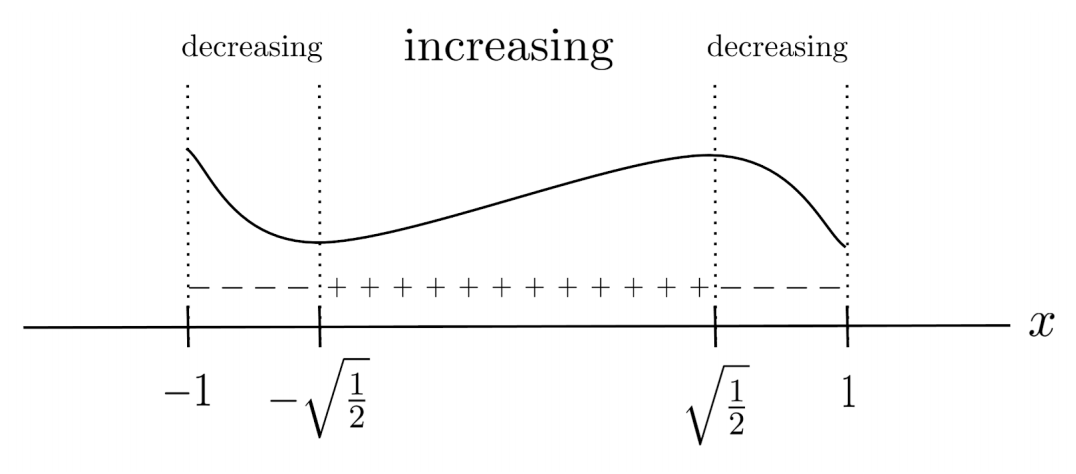## Exercises

For each function, find the critical points and label each critical point as a local maximum, local minimum, or saddle point. (You can view the solution by clicking on the problem.)

\begin{align*}1) \hspace{.5cm} f(x)= x^3-2x+1 \end{align*}
Solution:
\begin{align*} x &= -\sqrt{ \frac{2}{3} } \hspace{.5cm} \text{(max)} \\ x &= \sqrt{ \frac{2}{3} } \hspace{.5cm} \text{(min)} \end{align*}

\begin{align*}2) \hspace{.5cm} f(x)= x^4+10x^3-5 \end{align*}
Solution:
\begin{align*} x &= -\frac{15}{2} \hspace{.5cm} \text{(min)} \\ x &= 0 \hspace{.5cm} \text{(saddle)} \end{align*}

\begin{align*}3) \hspace{.5cm} f(x)= x \ln x \end{align*}
Solution:
\begin{align*} x &= \frac{1}{e} \hspace{.5cm} \text{(min)} \end{align*}

\begin{align*}4) \hspace{.5cm} f(x)= \ln(5x-x^2) \end{align*}
Solution:
\begin{align*} x &= \frac{5}{2} \hspace{.5cm} \text{(max)} \end{align*}

\begin{align*}5) \hspace{.5cm} f(x)= \frac{x^2+1}{x+1} \end{align*}
Solution:
\begin{align*} x &= -1-\sqrt{2} \hspace{.5cm} \text{(max)} \\ x &= -1+\sqrt{2} \hspace{.5cm} \text{(min)} \end{align*}

\begin{align*}6) \hspace{.5cm} f(x)= \frac{1}{x^2} e^{x^2-1} \end{align*}
Solution:
\begin{align*} x &= -1 \hspace{.5cm} \text{(min)} \\ x &= 1 \hspace{.5cm} \text{(min)} \end{align*}

\begin{align*}7) \hspace{.5cm} & f(x) = 4-x^3 \\ &x \in [-1,2] \end{align*}
Solution:
\begin{align*} x &= -1 \hspace{.5cm} \text{(max)} \\ x &= 0 \hspace{.5cm} \text{(saddle)} \\ x &= 2 \hspace{.5cm} \text{(min)} \end{align*}

\begin{align*}8)\hspace{.5cm} &f(x) = x^3 - 4x^2 \\ &x \in [-3,3] \end{align*}
Solution:
\begin{align*} x &= -3 \hspace{.5cm} \text{(min)} \\ x &= 0 \hspace{.5cm} \text{(max)} \\ x &= \frac{8}{3} \hspace{.5cm} \text{(min)} \\ x &= 3 \hspace{.5cm} \text{(max)} \end{align*}

\begin{align*}9) \hspace{.5cm} &f(x) = \frac{x^2}{x+1} \\ &x \in [-5,5] \end{align*}
Solution:
\begin{align*} x &= -5 \hspace{.5cm} \text{(min)} \\ x &= -2 \hspace{.5cm} \text{(max)} \\ x &= 0 \hspace{.5cm} \text{(min)} \\ x &= 5 \hspace{.5cm} \text{(max)} \end{align*}

\begin{align*}10) \hspace{.5cm} f(x) &= \frac{1+\ln x}{x} \\ &x \in \left[ \frac{1}{2}, 10 \right] \end{align*}
Solution:
\begin{align*} x &= \frac{1}{2} \hspace{.5cm} \text{(min)} \\ x &= 1 \hspace{.5cm} \text{(max)} \\ x &= 10 \hspace{.5cm} \text{(min)} \end{align*}

This post is part of a series.

Tags: